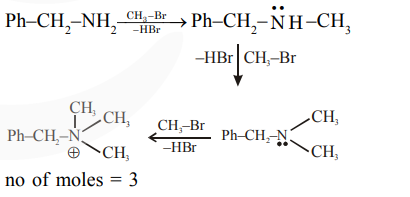# A reaction of 0.1 mole of Benzylamine with bromomethane`
Question:

A reaction of $0.1$ mole of Benzylamine with bromomethane gave $23 \mathrm{~g}$ of Benzyl trimethyl ammonium bromide. The number of moles of bromomethane consumed in this reaction are $\mathrm{n} \times 10^{-1}$, when $\mathrm{n}=$ . (Round off to the Nearest Integer).

(Given: Atomic masses: $\mathrm{C}: 12.0 \mathrm{u}$,

$\mathrm{H}: 1.0 \mathrm{u}, \mathrm{N}: 14.0 \mathrm{u}, \mathrm{Br}: 80.0 \mathrm{u}]$

Solution: